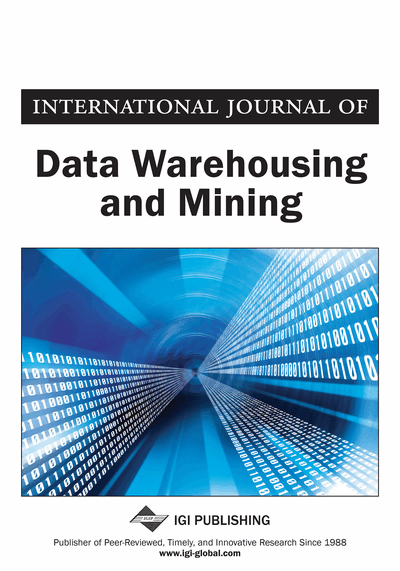# Weak Ratio Rules: A Generalized Boolean Association Rules

Baoqing Jiang (Henan University, China), Xiaohua Hu (Henan University China and Drexel Univeristy, USA), Qing Wei (Henan University of Economics and Law, China), Jingjing Song (Qingyuan Polytechnic, China), Chong Han (Henan University, China) and Meng Liang (Henan University, China)
DOI: 10.4018/jdwm.2011070103

## Abstract

This paper examines the problem of weak ratio rules between nonnegative real-valued data in a transactional database. The weak ratio rule is a weaker form than Flip Korn’s ratio rule. After analyzing the mathematical model of weak ratio rules problem, the authors conclude that it is a generalization of Boolean association rules problem and every weak ratio rule is supported by a Boolean association rule. Following the properties of weak ratio rules, the authors propose an algorithm for mining an important subset of weak ratio rules and construct a weak ratio rule uncertainty reasoning method. An example is given to show how to apply weak ratio rules to reconstruct lost data, and forecast and detect outliers.
Article Preview
Top

## Introduction

The problem of mining association rules from large databases has been subject of numerous studies. Some of them focus on developing faster algorithms for the classical method and/or adapting the algorithms to various situations, for example, distributed algorithm ODAM (Ashrafi, Taniar, & Smith, 2004), association rules mining in data warehouses (Tjioe & Taniar, 2005), multidimensional database mining (Yu et al., 2009; Casali et al., 2010), exception rules mining (Daly & Taniar, 2004; Taniar, Rahayu, Lee, & Daly, 2008) and redundant analysis (Ashrafi, Taniar, & Smith, 2007). Another direction is to define rules that modify some conditions of the classical rules to adapt to new applications (Marcus, Maletic, & Lin, 2001; Giannikopoulos, Varlamis, & Eirinaki, 2010). For instance, Srikant and Agrawal (1996) extended the categorical definition to include quantitative data and investigated the quantitative association rules problems.

For quantitative attributes, the general idea is partitioning the domain of a quantitative attribute into intervals, and applying Boolean algorithms to the intervals. But there is a conflict between the minimum support problem and the minimum confidence problem, while existing partitioning methods cannot avoid the conflict (Tong et al., 2005).

Fuzzy method had successfully been used in data mining (Kuok, Fu, & Wong, 1998; Kwok, Smith, Lozano, & Taniar, 2002). Fuzzy association rules solve interval partition problem in some extent. It needs to do fuzzy partition to the domain of quantitative attribute, i.e., to consider fuzzy set on the domain. However, in some actual problem, to some test data, we can not propose meaningful fuzzy sets.

Flip Korn et al. (1998) focused on real-valued data such as dollar amounts spent by customers on products in transactional database and proposed the following ratio rule:

which means that the ratio of the dollar amounts spent by a customer on bread to butter is. Flip Korn also investigated the application of ratio rule on data cleaning, forecasting, “what-if” scenarios, outlier detection and visualization.

When quantitative attributes have no ratio relation, ratio rule can not better describe the quantitative association relation of quantitative attribute. At this time, there not exist real number a, b such thatfor attributes x, y, but there may exist real number a, b such that.

In this paper, we focus on the relationship between nonnegative real-valued data in transactional database and investigate the influence of the values, of some attributes called antecedent, to the values of other attributes called consequent. When antecedent and consequent only include one attribute, the quantitative relationship of antecedent and consequent can be expressed as. For example,has such a reasoning meaning that if the dollar amount spent by a customer on bread is 2, then the dollar amounts spent by the customer on butter is at least 3. It is precisely this reason that the rules proposed by this paper are called weak ratio rules.

In order to establish the mathematical model of weak ratio rules problem, we investigate the Boolean association rules problem deeply and propose the equivalent description of it.

## Complete Article List

Search this Journal:
Reset
Open Access Articles
Volume 16: 4 Issues (2020): 2 Released, 2 Forthcoming
Volume 15: 4 Issues (2019)
Volume 14: 4 Issues (2018)
Volume 13: 4 Issues (2017)
Volume 12: 4 Issues (2016)
Volume 11: 4 Issues (2015)
Volume 10: 4 Issues (2014)
Volume 9: 4 Issues (2013)
Volume 8: 4 Issues (2012)
Volume 7: 4 Issues (2011)
Volume 6: 4 Issues (2010)
Volume 5: 4 Issues (2009)
Volume 4: 4 Issues (2008)
Volume 3: 4 Issues (2007)
Volume 2: 4 Issues (2006)
Volume 1: 4 Issues (2005)
View Complete Journal Contents Listing